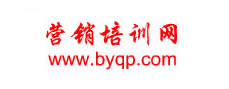成立于2002最专业营销培训销售培训团队管理服务商　　　　　　　全国统一咨询热线：010-51652313 13681285133 862869709                 | 旧版回顾设首页 加收藏您当前的位置：中华营销培训网 > 友情连接
<%If FlushAddress <> 1 Then%> <%End If%> <%If FlushAddress <> 1 Then%> <%End If%> <%If FlushAddress <> 1 Then%> <%End If%> <%If FlushAddress <> 1 Then%> <%End If%> <%If FlushAddress <> 1 Then%> <%End If%> <%If FlushAddress <> 1 Then%> <%End If%> <%If FlushAddress <> 1 Then%> <%End If%> <%If FlushAddress <> 1 Then%> <%End If%> <%If FlushAddress <> 1 Then%> <%End If%> <%If FlushAddress <> 1 Then%> <%End If%> <%If FlushAddress <> 1 Then%> <%End If%> <%If FlushAddress <> 1 Then%> <%End If%> <%If FlushAddress <> 1 Then%> <%End If%> <%If FlushAddress <> 1 Then%> <%End If%> <%If FlushAddress <> 1 Then%> <%End If%> <%If FlushAddress <> 1 Then%> <%End If%> <%If FlushAddress <> 1 Then%> <%End If%>

 共有友情连接 17 个  首 页  上一页   下一页  尾 页  页次：1/1页  20个/页
 关于本站 - 网站帮助 - 广告合作 - 下载声明 - 友情连接 - 网站地图
 Copyright@2002-2014 www.byqp.com,All Rights Reserved. 京ICP备12042043号 京公网安备11010802011457号 本站网络实名：中华营销培训网 本站域名：www.byqp.com 公司地址：北京大学燕园 法律顾问：北京中仁律师事务所 　 律师：马国华 　电话：（010）88554220 88554219 全国统一咨询热线：010-51652313　13681285133 客服Email： byqpwin@126.com：